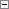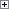Calculates a checksum for a string that contains a number.

CheckNumber :=STRCHECKSUM(String[, WeightString][, Modulus])

Parameters

String

Type: Text constant or code

This string contains the number for which you want to calculate a checksum. You can only enter the numeric characters 0-9 in this string. If you enter anything else, a run-time error will occur. If String is empty, 0 is returned.

WeightString

Type: Text constant or code

This string contains numbers that you want to use as weights when calculating the checksum. The default value is a string that contains STRLEN(String) '1'-characters.

You can only enter the numeric characters 0-9 in this string. If you enter anything else, then a run-time error occurs.

If String is longer than WeightString, then a string is concatenated that contains STRLEN(String) - STRLEN(WeightString) '1'-characters to the end of WeightString. If WeightString is longer than String, then a run-time error occurs.

Modulus

Type: Integer

The number that you want to use in the checksum formula. The default value is 10.Property Value/Return Value

Type: Integer

The checksum, which is calculated using this formula:Example

This example shows how to use the STRCHECKSUM function to calculate a checksum.

This example requires that you create the following variables and text constants in the C/AL Globals window.

Variable name DataType Length

StrNumber

Text

30

Weight

Text

30

Modulus

Integer

Not applicable

Text constant ENU value

Text000

The number: %1\

Text001

has the checksum: %2Copy Code
StrNumber := '4378';
Weight := '1234';
Modulus := 7;
CheckSum := STRCHECKSUM(StrNumber, Weight, Modulus);
MESSAGE(Text000 + Text001, StrNumber, CheckSum);

The formula is:

(7 - (4x1 + 3x2 + 7x3 + 8x4) MOD 7) MOD 7=0

The message window displays the following:

The number: 4378

has the checksum: 0Example

This example shows how to use the STRCHECKSUM function to calculate a modulus 10 checksum for a bar code.

The STRCHECKSUM function can be used to calculate checksums for 13- and 8-digit European Article Number (EAN) and EAN-compatible bar codes such as a Universal Product Code (UPC) or Japanese Article Number (JAN).

A 13-digit EAN code has the following format:

1. The 12 digits in positions 13 to 2 are used to calculate the checksum at position 1.
2. Starting with position 2, all even values are totaled. The result is then multiplied by three. This value is called Even.
3. Starting with position 3, all odd values are totaled. The result is called Odd.
4. Total=Even + Odd.
5. The modulus 10 checksum is then (10 - Total MOD 10) MOD 10.

This example requires that you create the following variables and text constants in the C/AL Globals window.

Variable name DataType Length

StrNumber

Text

30

Weight

Text

30

Modulus

Integer

Not applicable

Text constant ENU value

Text000

The EAN code: %1\

Text001

has the checksum: %2Copy Code
StrNumber := '577622135746';
Weight := '131313131313';
CheckSum := STRCHECKSUM(StrNumber, Weight);
MESSAGE(Text000 + Text001, StrNumber, CheckSum);

The message window displays the following:

The EAN code: 577622135746

has the checksum: 3

Code Data Type
Text Data Type